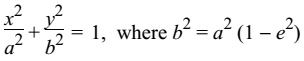# Standard Equation of Ellipse

The locus of a point in a plane whose distance from a fixed point bears a constant ratio, less than one to its distance from a fixed line is called ellipse.It is symmetrical about x-axis and y-axis simultaneously and hence about the origin. It does not pass through the origin.

The standard equation of an ellipse is: